## A Method for Factoring that Often Works

Suppose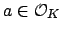is such that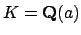, and let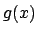be the minimal polynomial of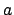. Then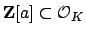, and we have a diagram of schemes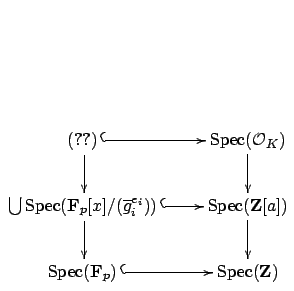where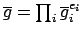is the factorization of the image of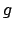in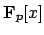.

The cover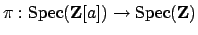is easy to understand because it is defined by the single equation. To give a maximal ideal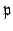of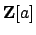such that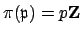is the same as giving a homomorphism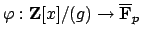(up to automorphisms of the image), which is in turn the same as giving a root ofin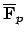(up to automorphism), which is the same as giving an irreducible factor of the reduction ofmodulo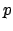.

Lemma 8.1.2   Suppose the index ofin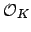is coprime to. Then the primes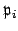in the factorization of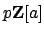do not decompose further going fromto, so finding the prime ideals ofthat containyields the factorization of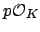.

Proof. Hi-brow argument: By hypothesis we have an exact sequence of abelian groups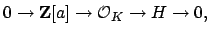where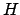is a finite abelian group of order coprime to. Tensor product is right exact, and there is an exact sequence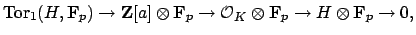and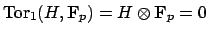, so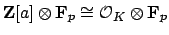.
Low-brow argument: The inclusion map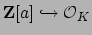is defined by a matrix over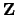that has determinant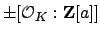, which is coprime to. The reduction of this matrix modulois invertible, so it defines an isomorphism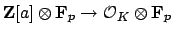. Any homomorphism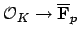is the composition of a homomorphism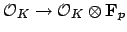with a homomorphism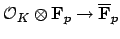. Since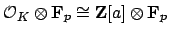, the homomorphismsare in bijection with the homomorphisms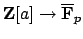, which proves the lemma.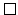As suggested in the proof of the lemma, we find all homomorphismsby finding all homomorphism. In terms of ideals, if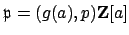is a maximal ideal of, then the ideal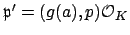ofis also maximal, since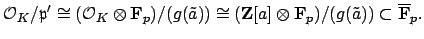We formalize the above discussion in the following theorem:

Theorem 8.1.3   Let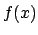denote the minimal polynomial ofover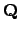. Suppose that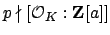is a prime. Let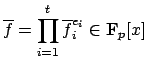where the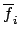are distinct monic irreducible polynomials. Let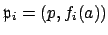where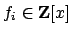is a lift ofin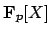. ThenWe return to the example from above, in which, whereis a root of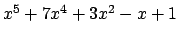. According to , the maximal orderhas discriminant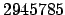:

   > Discriminant(MaximalOrder(K));
2945785

The orderhas the same discriminant as, so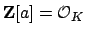and we can apply the above theorem.
   > Discriminant(x^5 + 7*x^4 + 3*x^2 - x + 1);
2945785

We have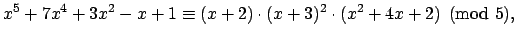which yields the factorization of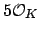given before the theorem.

If we replaceby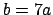, then the index of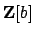inwill be a power of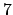, which is coprime to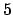, so the above method will still work.

   > f:=MinimalPolynomial(7*a);
> f;
x^5 + 49*x^4 + 1029*x^2 - 2401*x + 16807
> Discriminant(f);
235050861175510968365785
> Discriminant(f)/Discriminant(MaximalOrder(K));
79792266297612001    // coprime to 5
> S<t> := PolynomialRing(GF(5));
> Factorization(S!f);
[
<t + 1, 2>,
<t + 4, 1>,
<t^2 + 3*t + 3, 1>
]

Thusfactors inas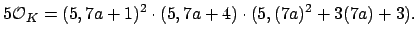If we replaceby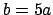and try the above algorithm with, then the method fails because the index ofinis divisible by.
   > f:=MinimalPolynomial(5*a);
> f;
x^5 + 35*x^4 + 375*x^2 - 625*x + 3125
> Discriminant(f) / Discriminant(MaximalOrder(K));
95367431640625    // divisible by 5
> Factorization(S!f);
[
<t, 5>
]


William Stein 2004-05-06